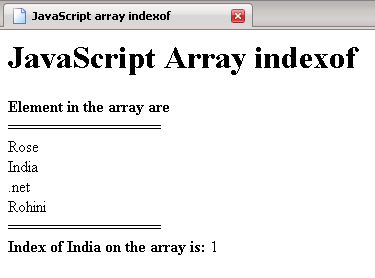Tutorials

# JavaScript array index of

In this Tutorial we want to describe that makes you to easy to understand JavaScript array index of.

In this Tutorial we want to describe that makes you to easy to understand JavaScript array index of.

# JavaScript array index of

In this Tutorial we want to describe that makes you to easy to understand JavaScript array index of. We are using JavaScript as scripting language. An array var instantiate array object that hold the values of an elements. The document. write print the Element in the array are followed by break line. The for loop execute the script till variable  i is less than the length of an array. The document. write print the value of element that an array object hold specified by - array indexing followed by break line.

1)index of( )  - This return the position of the value that is hold by an array object and store in a variable index.

Finally the document. write print the index position of India on array.

JavaScript_array_indexof.html

 ```

JavaScript Array indexof

JavaScript array indexof                var array=new Array();   array="Rose";   array="India";   array=".net";   array="Rohini";   document.write(""+"Element in the array are "+""+ "");   document.write("================="+ "");   for(var i=0;i")   }   document.write("================="+ "");   var index = array.indexOf("India");  document.write(""+"Index of India on the array is: "+""+index);        ```

Output of the program